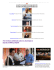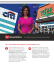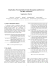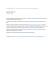# CNN optimizations for embedded systems and FFT

## Transcription

CNN optimizations for embedded systems and FFT
```CNN optimizations for embedded systems and FFT
Artem Vasilyev
Stanford
353 Sierra Mall Stanford CA 94305
[email protected]
both approaches at the same time and co designing
algorithms and hardware at the same time.
Abstract
2. Problem statement
CNNs have proven to be a very successful yet
computationally expensive technique which made them
slow to be adopted in mobile and embedded systems.
There is a number of possible optimizations:
minimizing the memory footprint, using lower precision
and approximate computation, reducing computation cost
of convolutions with FFTs. These have been explored
recently and were shown to work.
This project take ideas of using FFTs further and
develops an alternative way to computing CNN – purely in
frequency domain. As a side result it develops intuition
about nonlinear elements: why do they work and how new
types can be created.
In specialized hardware, most of the gains are archived
by select “the right” algorithm – the one which can be
implemented efficiently.
For embedded platform the measure of efficiency is
power which is equal to number of operations times
energy per operations. To optimize power we can either do
less operations or spend less energy doing it.
2.1. Energy table
First of all it‟s important to understand how the energy
is spent on various steps in computer program.
Operation
16b Int MULT
16b FP MULT
32b FP MULT
Register File, 1kB
L1 Cache, 32kB
L2 Cache, 256kB
on-chip DRAM
DRAM
Wireless transfer
1. Introduction
Today mobile devices such as smartphones and tables
are the most often used compute platform and digital
camera as such they are the best target for various
computer vision applications. These devices are battery
powered which limits available resources and poses a
significant challenge to advanced algorithms such as
CNN.
Traditionally CNNs have been developed for clusters of
and 200W respectively, while mobile platforms are limited
at 5-10W. This gets even worse for wearable and “always
on” devices which only have 0.1-1W.
A common approach to alleviate performance and
power problem (to some degree) is to move computation
to “the cloud”, however this raises privacy concerns about
doesn‟t solve the power problem if we want to apply
CNNs in real time. Sending even small 256x256 images at
25fps over the data network will quickly drain the battery,
not to mention huge amounts of latency.
The only way to solve this problem is by building
specialized hardware such as NeuFlow or
algorithm to better fit existing hardware and it‟s
limitations. The best results could be archived by applying
Energy, pJ
0.06
0.8
0.45
1.1
1.0
4.5
0.6
3.5
30.2
160
640
60000
Relative cost
1
13
8
18
17
80
10
58
500
2667
10667
1000000
Table 1: Energy cost of common operations.
Numbers in Table 1 heavily depend on technology
parameters, such as manufacturing node (feature size),
operating voltage, frequency, etc. but the general trend
will be same:
 communication is extremely expensive
 computation is cheaper than memory access
 memory access depends on it‟s capacity
(register file vs cache vs DRAM)
 integer arithmetic is cheaper than floating point
1

And the computation increase can be offset by:
 Interger/Fixed point arithmetic instead of floats
 Aproximate/Imprecise computation
 Use less precision / fewer bits
 Do convolutions in frequency domain
everything depends on data precision(16b vs
32b)
This clearly shows the importance of data locality and
memory footprint optimization to energy efficiency.
2.2. Choosing CNN implementation
All these ideas have merit and there are very recent
papers ( from 2015) that explored them, however those
papers used old CNN designs as a baseline link
AlexNet, OverFeat or Maxout, no paper have
considered GoogLeNet, but in general the same
methods will likely work.
For a baseline architecture in embedded system we‟d
want to use one of the top performing submitions in
ImageNet challenge, that has the minimal working set. In
other words, the CNN with smallest number of
parameters.
CNN
AlexNet
Clarify
OverFeat
VGG 
Year
2012
2013
2013
2014
2014
Parameters
60M
65M
70M
135M
7M
3.1. Setting some weights to 0
The main assumption is that some weights are less
important than others and can be set to 0 and that this will
have small to moderated affect on the accuracy.
M. D. Zeiler and R. Fergus showed that the
convolotional layers gradually increase the accuracy of
CNN which supports my assumptions. Furthermore
techniques like DropConnect  suggest that remove
some parameters is not only acceptable, but could also be
beneficial and certain regularizations types are also
known to encourage sparse parameters as shown in
Elastic Net .
Table 2: Number of parameters in state-of-the-art CNNs.
As the summary table 2 shows, in general, the trend has
been towards increasing the number of weights. This is
cause by increasing complexity of CNN and adding more
layers.
An exception to this trend is 2014 winner – GoogLeNet.
By comparing it with VGG, we can notice that there are
more convolution layers – 59 stages of varying sizes in 21
layers vs 16 stages of 3x3 convolutions over 16 layers in
less parameters because there is a one instead three Fully
Connected layers.
Even though such a reduction is great we are still need
7m*4Byte=28MB of storage this is still too high from
SRAM and would require DRAM access. Additionally we
are doing about 3x more computations (59 vs 21
convolution stages).
GoogLeNet use a lot of convolutions, in fact it spends
majority of computations doing them. For this reason it‟s
critical to have a very efficient implementation of
convolution. One of the options is by using Fast Fourier
Transformation. This will turn convolutions into pointwise multiplications and reduce complexity from O(n2*k2)
to O(n2) where n is the input width/height and k is the
filter size. Section 4 describes how this idea can be
developed further.
3.2. Quantizing weights
L2 is most commonly used regularization type it
penalizes large values in weights and encourages that all
parameters are used a little. This suggest that we can
expect relatively small dynamic range in weights thus use
smaller number of bits to represent them.
3.3. Weights deduplication
Data deduptication is a well know technique to reduce
memory requirement. It doesn‟t change the data and thus
will not affect the accuracy and it can be efficiently
integrated into computer architecture as was shown by
HICAMP.
The simplest way to simulate the benefits of
deduplication is by using compression, like Zip.
3.4. Lower precision arithmetic
O. Temam  investigated neural network hardware
accelerator geared towards defect tolerance and energy
efficiency. This is a very desirable feature of CNN which
allows cheaper and more efficient hardware
implementations. The fault tolerance can be investigated
in software by artificially injecting random errors during
computation. It seem that the fault tolerance could the
consequence of using more precision than required by the
algorithm.
3. Overview of previously explored ideas
The remaining problem with the number of parameters
can be addressed by the following optimizations:
 Setting some weights to 0
 Quantizing weights to fewer bits
 Weights Deduplication
2
Courbariaux and David investigated the use of low
precision arithmetic for deep learning in . Their result
shows that Maxout architecture can use only 10bits for
computation and 12bits storage without significantly
affecting the accuracy.
S. Gupta et al.  also successfully used lower
precision arithmetic both for CNN and fully connected
architecture. Their paper used very simple CNN with 2
layers and small data set like CFAIR10, but they showed
that it‟s possible to use none standard 12bit float instead of
32bit single precision with small effect on the accuracy.
They report archiving energy efficiency of 37
GOps/second/W with low precision implementation in
fpga vs 1-5 GOps/second/W achievable on CPU/GPUs (
Intel i7-3720QM, NVIDIA GT650m and the GTX780)
4.2. Linear system analysis
A common way to analyze and work with linear systems
is through the use of a transfer function. A complicated
system can be broken into simple stages, each stage is
modifying a spectrum according to the transfer function
and feeds the output to the next stage.
Such method can‟t be directly applied to CNN because
of none linearity. Switching between time and frequency
domain (as in  and ) is one way to address it. The
advantage of this approach is that it can deal with all types
on none linear functions, but at the expense of doing iFFT
before none linearity and FFT after.
Alternatively we can use the properties of a particular
none linearity function and stay in the frequency domain.
Section 4. 5 will develop mathematic justification for the
case of ReLu() function which allows to treat it almost like
a linear system.
3.5. FFTs
A well know property of FFT is that it turns convolution
into element wise multiplication. Not only this requires
significantly less operations to compute, but it also
eliminates reeducation step in convolution (summation)
and thus exposes extra level of parallelism. This is a very
desirable characteristic in every parallel system, but
especially in GPUs which are optimized for fully
independent threads. Not surprisingly FFTs were used for
GPU optimization, fist by Mathieu et al. who reported
up to 3 times faster performance and in resent for of N.
Vasilache et al which archived 1.4 to 14.5 time better
performance than cuDNN by custom implementation of
FFTs tuned to small kernel sizes.
4.3. Convolution layer
As was mentioned before, convolution in frequency
domain becomes an element wise multiplication of the
Fourier components.
To perform element wise multiplication, the two arrays
must be of equal size, so it might seem that we have
increase the number of parameters from k2 to n2. But in
fact all n2 Fourier coefficients can be expressed through
k2 original parameters because we can view the coefficient
as a weighted sum of 2D delta functions:
𝑖,𝑗
𝛿 𝑥 − 𝑥𝑖 , 𝑦 − 𝑦𝑗 ∗ 𝑤(𝑖, 𝑗)
Delta function is defined over entire n2 domain, and it‟s
spectrum is known.
4. CNNs with FFTs
4.1. Problem statement and related work
4.4. Pooling layer
Works of Mathieu et al. and Vasilache et al are the
most related papers to my project. Both papers used
traditional CNN structure and interpretation of weights,
which means there have to do FFT, element wise
multiplication and inverse FFT on every convolution layer.
Both FFT and iFFT are O(n2log n) operations ( vs O(n2)
for element wise multiplication ). This greatly reduces the
benefits especially for small filters (see  for more
details).
My idea is to comute FFT only once on the input image
and do iFFT after the last convolution layer (or at the very
end). Modern CNNs like VGG and GoogLeNet
have up to 59 convolution stages in 21 layer and the
savings would be big, but it would also require doing both
NonLinearity and Pooling layers in frequency domains.
The next section develops mathematic framework to
approach this problem and reports experimental results for
Pooling layer and Nonlinearity.
Pooling layer can be done ether with MAX operation or
AVERAGE. This is the same as image decimation – a
standard practice image processing, it is well described by
Bouman in .
Decimation is equivalent to image blur, which removes
high frequencies for an image followed by size reduction.
This can be done in frequency domain by doing
convolution and discarding extra frequency components.
Figure 1: Pooling Layer in Frequency domain
3
MAX and Average are special kinds of blur kernels
used for their computation efficeincy (in space domain).
The later one is also known as box filter. Both are actually
considered suboptimal compared to 2D sinc function 
which better suppresses aliacing.
The comparison beween space and frequency domain
implementation is shown on Figure 1. It should be noted
that in frequency domain using box filter intead of sinc
doesn‟t give any benefits because both are just element
wise multiplications.
Figure 2 illustrates the method of computing ReLu in
frequency domain. We can see that the result is very close,
but not identical. The power spectrum plots indicate that
4.5. Nonlinearity in frequency domain
Any stage in the system can be viewed as applying a
certain function g(y) to the input function f(x),so analysis
in the frequency domain comes to finding the Fourier
transform F(g(f(x)) with respect to F(f(x)).
In general problem doesn‟t have an analytical solution,
but we can find one in case g(y)=ReLu(y)
The most common way none linearity is ReLu, which
acts as data clipping in time domain. It creates sharp
corners in the signal, so in the frequency domain this
would add higher frequency harmonics to the spectrum.
Mathematically we can express ReLu(f(x)) function
through f(x) as a multiplication with the sign(f(x)) : which
is equal to 1 if f(x)>0 and 0 otherwise :
𝑅𝑒𝐿𝑢 𝑓 𝑥
= max 𝑓 𝑥 , 0 = 𝑠𝑖𝑔𝑛 𝑓 𝑥
the difference comes from the edges (high frequency) and
is likely caused by limited image dimensions.
∗ 𝑓(𝑥)
4.6. Fully connected layer
Fully connected layer is a special case of convolution
layer where the result is computed for a single point. As
such, it turns into element wise multiplication in frequency
domain.
Typically, the input to this layer is very deep, but
narrow and short. But batching multiple image we can
make it wider and taller to increase the computation
efficiency.
Because we are working with limited intervals (number
of samples) of function f(x), we can express ReLu through
the multiplication with sum of delta functions:
𝑠𝑖𝑔𝑛 𝑓 𝑥
∗𝑓 𝑥 =𝑓 𝑥 ∗
𝛿 𝑥 − 𝑥𝑖 , 𝑓 𝑥𝑖 > 0
𝑖
The Fourier transform of a delta function is given by:
𝐹 𝛿 𝑥 − 𝑥0 (𝑘) = 𝑒 2𝜋𝑗𝑘 𝑥 0
4.7. Softmax
Using linearity of FFTs and convolution theorem we
can express the Fourier transform of ReLu(f(x)) through
the Fourier transform of f(x):
Softmax takes a vector as an input, but in frequency
domain every element of this vector is spread among all
frequencies. So we would need to use a matrix as an input
to softmax, or convert back to special domain with iFFT.
𝑒 2𝜋𝑗𝑘 𝑥 𝑖 ⨂𝐹 𝑓 𝑥
𝐹 𝑅𝑒𝐿𝑢(𝑓 𝑥 ) (𝑘) =
𝑖
4.8. Computational complexity
This shows that in frequency domain, ReLu() acts as a
convolution with the function of known form. However,
this function depends on the input and we need to find
positions in space domain 𝑥𝑖 : 𝑓 𝑥𝑖 > 0. To do that we
need to take inverse transforms of the input and solve the
inequality.
The key factor is that once we have found 𝑥𝑖 , we know
the transfer function of the ReLu for this input and don‟t
need to calculate FFT.
Figure 2: ReLu in Frequency domain
Since ReLu is equivalent to convolution in frequency
domain it might seem that we haven‟t gained anything by
using FFTs.
This is not the case because pooling in frequency
reduces the data by discarding elements after a certain
index. As a result, the convolution doesn‟t need to
compute that data and has to generate only ¼ of the points.
Overall the complexity of the algorithm seems to be:
4
O(n2log n) vs O(n2 k2) in space domain. However, the
constant factor and lower order terms seems to be smaller.
Additionally, authors of  showed that the benefit of
independent operation in element by element
multiplication. It maps well on modern SIMD architecture
and runs faster, however a careful code tuning is required
to take full advantage of this property
Because asymptotically O(n2log n) is worse than O(n2
2
k ), we can expect that there is a point where two methods
run in the same time.
4.9. Putting it all together
For the final experiment, I have implement all layers
python, such that they interface with each other by passing
frequency activations instead of special ones.
This required soling a number of issues like: in
dimensions are even the location of 0 fequency in the
array is not obvious (different form MatLab), all
frequencies and spectrum have to account the number of
samples, etc.
However the one issue worth noting is that in
frequency domain, just like in space domain, we have to
deal with the boundary conditions by using more elements
than in the incoming activations. In special domain this
was a simple padding with 0, and in frequency domain this
requires changing all elements of array (because in numpy
implementation frequency depends on the number of
samples).
In the end my implantation ran much slower compared
to optimized computations we used in homework‟s and I
was getting poor prediction quality because the weights
were trained on a traditional network in space domain and
then transferred over to frequency CNNs, which doesn‟t
not compute identical result due to boundary conditions
and other implementation details hinted earlier.
Figure 3 illustrate this on a single block of three
consecutive operations: Convolution, ReLu, Pool. An
input was 151 by 151„Lenna‟ image and Sobel filter
operator: [[-1,-2,-1],[0,0,0],[1,2,1]].
We can see that even though the error on each layer are
small (as shown in Fig.1 and 2), they accumulate and they
are not concentrated in a small or not important area of the
image.
This issue will likely be corrected by using the same
frequency computations during training, instead of doing
them only during testing. Also we can back propagate
purely in the frequency domain – we are doing same kinds
of operations – multiplications, additions and
convolutions.
Figure 3: Conv-ReLu-Pool – error accumulation frequency
domain
Nonlinear elements are often described as “magic” that
makes CNN works. They are given as the reason why
CNNs can express “interesting” functions that separate
object classes, but very little is given as explanation of
their mechanics other that the fact that ReLus “seem to
perform better than others”.
We have seen that ReLu acts as convolution in
Frequency domain. Let‟s try to develop an intuition about
other common types: sigmoid and tanh by looking at their
modification to a spectrum of function sin(50x).
In case of a linear system, we‟d simply derived a
transfer function by applying a step function as an input.
But since the system is not linear, we can only develop
intuition without mathematical backing.
As we can see from Figure 4, all three act similarly by
“spreading” information from a single frequency band at
50Hz to other harmonics: 2x and 4x in case of ReLu and
3x in case of sigmoid and tanh. Also it‟s clear that ReLu
redistributes information better – the peak at 2x is 20% of
the oginal, while sigmoid only 20% at the original location
and a tiny portion at 3x, putting most of the energy in DC.
High emphasis of nonlinearity on DC component is bad
because all the frequency would be smashed into the same
bin and become indistinguishable.
We also know that ReLu is followed by Pooling which
discards some frequency components, so a more even
redistribution has a better chance of preserving some of
the informational content from the frequency that will be
5
6. Conclusion and future works
In this project I did a review of possible methods to
optimize CNN for embedded platform and developed an
new way to perform all the computation in frequency
domain. This method exposed an intriguing “duality” of
CNN: a convolution operation is require both in special
domain and in frequency domain, however they have
different purpose and meaning. Convolution in space
captures special locality in the data, while convolution in
frequency redistributes information to different
components in order to mitigate information loss in the
Pooling layer.
The goal of doing computation in frequency domain
was to eliminate convolutions, while it‟s impossible to do
without constantly switching between space and frequency
(which is expensive), computation entirely in frequency
domain has to calculate ¼ of convolution results compared
to special domain.
The project has demonstrated that this is a viable
approach but computation has to be done the same way
during training and testing. We also saw that it‟s possible
to match the exact behavior of ReLu, but it‟s
computationally expensive. However this is probably not
required for the successful operation and other
nonlinearities are possible.
A good follow up would be to explore different kinds of
nonlinearities by performing convolutions in space, a more
optimized implementation similar to work in  and
performing training in frequency domain.
Figure 4: Effect of none linearity
References
 M. Mathieu, M. Henaff and Y. LeCun. Fast training of
convolutional networks through ffts. CoRR, 2014
 N. Vasilache, J. Johnson, M. Mathieu,S. Chintala, S.
Piantino and Y. LeCun, Fast Convolutional Nets With fbfft
:A GPU Performance Evaluation. Under review at ICLR,
http://arxiv.org/abs/1412.7580, Under review at ICLR,2015
 A. Krizhevsky, I. Sutskever, and G. Hinton. ImageNet
classification with deep convolutional neural networks.
NIPS, 2012.
 Goodfellow, I. J., Warde-Farley, D., Mirza, M., Courville,
A., and Bengio, Y. Maxout networks. Technical report,
2013
 P. Sermanet, D. Eigen, X. Zhang, M. Mathieu, R. Fergus,
and Y. LeCun. Overfeat: Integrated recognition,
localization and detection using convolutional networks.
ICLR, 2014.
 C. Szegedy, W. Liu, Y. Jia, P. Sermanet, S. Reed, D.
Anguelov, D. Erhan, V. Vanhoucke, A. Rabinovich. Going
Deeper with Convolutions. http://arxiv.org/abs/1409.4842.
2014
 http://www.clarifai.com/
 K. Simonyan, A. Zisserman. Very Deep Convolutional
Networks
for
Large-Scale
Image
Recognition.
http://arxiv.org/abs/1409.1556, Under review at ICLR,2015
5.1. Generating new nonlinearities
As we saw, ReLu, sigmoid and tanh act similarly, by
redistributing information for original image, we can
design new kind of functions to do the same thing.
A possible strategy is to use convolutions in frequency
domain (just like ReLu does), but employ different kenrels
than ReLu. And important feature is that the kernel
applied to each activation has to depend on that
activation, in other words it‟s not constant. ReLu bases the
kernel on mask which translates to a large kernel in
frequency domain, but we can use smaller kernel and even
one based on frequencies only instead of information from
special domain. We can also create one that doesn‟t
emphases DC component.
Of cause, we would have to train the network differently
and do a proper back propagation.
6
 M. D. Zeiler and R. Fergus. Visualizing and Understanding
Convolutional Networks. CoRR, 2013
 L. Wan et al. Regularization of Neural Networks using
DropConnect. ICML, 2013
 H. Zou ,T. Hastie, Regularization and Variable Selection via
the
Elastic
Net.
http://web.stanford.edu/~hastie/Papers/elasticnet.pdf, 2003
 M. Courbariaux and J.-P. David. Low precision arithmetic
for
deep
learning.
Under
review
at
ICLR,
http://arxiv.org/abs/1412.7024 , 2015
 S. Gupta, A. Agrawal, K. Gopalakrishnan P. Narayanan.
Deep Learning with Limited Numerical Precision,
http://arxiv.org/pdf/1502.02551v1.pdf ,2015
 D. Cheriton, A. Firoozshahian, A. Solomatnikov, J. P.
Stevenson, O. Azizi. HICAMP: architectural support for
efficient concurrency-safe shared structured data access,
http://dl.acm.org/citation.cfm?id=2151007, ACM, 2012
 C. Farabet, B. Martini, B. Corda, P. Akselrod, E.
Culurciello, and Y. LeCun. Neuflow: A runtime
reconfigurable dataflow processor for vision, in Proc.
Embedded Computer Vision Workshop, 2011.
 O. Temam. A Defect-Tolerant Accelerator for Emerging
High-Performance Applications. ISCA, 2012
 Y. Chen, T. Luo, S. Liu, S. Zhang, L. He, J. Wang, L. Li, T.
Chen, Z. Xu, N. Sun, and O. Temam. DaDianNao: A
Machine-Learning Supercomputer. MICRO'14, 2014
 Y. Jia. Caffe: An open source convolutional architecture for
fast feature embedding. http://caffe.berkeleyvision.org/,
2013.
 P. Warden. How to run the Caffe deep learning vision
library on Nvidia‟s Jetson mobile GPU board,
http://petewarden.com/2014/10/ , 2014
 Theano, http://deeplearning.net/software/theano/, 2015
 Torch, http://torch.ch/, 2015
 Pylearn2, http://deeplearning.net/software/pylearn2/, 2015
 Y. Jia, E. Shelhamer, J. Donahue, S. Karayev, J. Long, R.
Girshick, S. Guadarrama, T. Darrell. Caffe: Convolutional
Architecture
for
Fast
Feature
Embedding.
http://arxiv.org/abs/1408.5093 , 2014
 Caffe
Model
Zoo
,
http://caffe.berkeleyvision.org/model_zoo.html , 2015
 C. A. Bouman. Digital Image Processing .
https://engineering.purdue.edu/~bouman/ece637/notes/pdf/
RateConversion.pdf, 2015
 Fourier transform.
http://en.wikipedia.org/wiki/Fourier_transform , 2015
7
```

### About CNN Newsource Live Services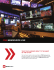### AlexNet - Stanford Vision Lab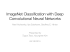### Convolutional Neural Networks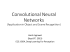### 175 Sandy stories with more than 200 images At the Height of the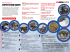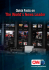### New-Yorkers-celebrat..